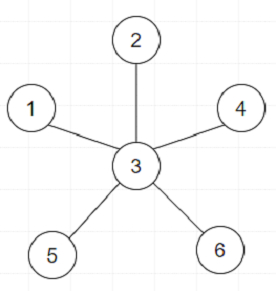# Program to find center of star graph in Python

PythonServer Side ProgrammingProgramming

Suppose we have one undirected star graph with n nodes labeled from 1 to n. As we know a star graph is a graph where there is one center node and exactly n - 1 edges that connect the center node with every other node. We have to find the center of the given star graph.

So, if the input is likethen the output will be 3 as 3 is at center.

To solve this, we will follow these steps −

• seen := a new set

• for each edge (u,v) in graph, do

• if u is in seen, then

• return u

• if v is in seen, then

• return v

• insert u into seen

• insert v into seen

## Example

Let us see the following implementation to get better understanding −

def solve(graph):
seen = set()

for u,v in graph:
if u in seen:
return u
if v in seen:
return v

graph = [(1,3),(2,3),(4,3),(5,3),(6,3)]
print(solve(graph))

## Input

[(1,3),(2,3),(4,3),(5,3),(6,3)]


## Output

3